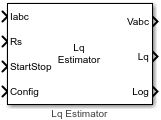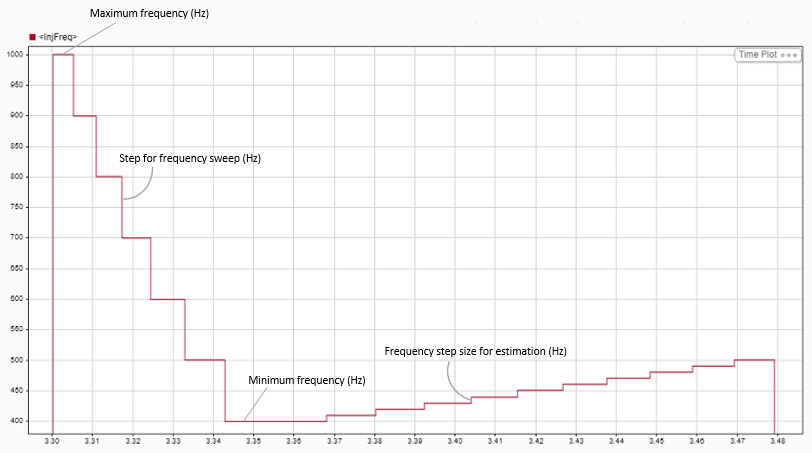# Lq Estimator

Estimate stator inductance (Lq) of permanent magnet synchronous motor (PMSM)

Since R2023a

•Libraries:
Motor Control Blockset / Parameter Estimation / PMSM Parameter Estimation

## Description

The Lq Estimator block computes the q-axis stator inductance of a PMSM using the phase currents, stator resistance, and the test configuration elements.

At first, the block locks the rotor along the d-axis by injecting a constant voltage (Vd), which is configurable using the parameter Vdq bias voltage (PU) of the PMSM Parameter Estimation Configurator block. This ensures that the rotor remains stationary (aligned with d-axis) during frequency injection. To estimate q-axis stator inductance, the block injects a high-frequency voltage signal along the q-axis and then performs the frequency sweep process by gradually reducing the injected signal frequency using the step size defined by Step for frequency sweep (Hz) parameter. During frequency sweep, the block monitors the magnitude of the motor phase current. When the current magnitude becomes appropriately high or when the injected signal frequency reaches the minimum value (Minimum frequency (Hz) parameter), the block starts the estimation process by injecting a signal with rising frequency having a step size of Frequency step size for estimation (Hz) with number of steps defined by the Number of frequencies for estimation parameter. For details about these parameters, see PMSM Parameter Estimation Configurator.

This image shows the frequency sweep and the incremental steps of rising frequency.The Lq estimation process uses the measured frequency sweep time duration as well as the step size of the changing frequency to estimate the q-axis stator inductance, Lq.

## Ports

### Input

expand all

The three-phase current response of the motor measured by the current sensors.

Note

Providing phase-c current input is optional. The block functionality is not impacted if you do not provide the phase-c current input.

Data Types: `single`

Estimated stator resistance of the PMSM. You can either connect this input port to the `Rs` output of the PMSM Rs Estimator block or provide the resistance value manually using a Constant block.

Data Types: `single`

Signal that either starts or stops Lq estimation:

• `1` (rising edge of a pulse) — This signal initiates the Lq estimation.

• `0` (falling edge of a pulse) — This signal immediately stops the Lq estimation process. If you interrupt the estimation process abruptly before completion, the block does not compute or output the Lq value.

Data Types: `Boolean`

This table summarizes the expected configuration elements in the bus signal input.

CategoryConfiguration elementUnits

Elements for motor and inverter hardware

Number of pole pairs

-

Rated speed of motor

RPM

Rated current of motor

A

Maximum measurable inverter current

A

Board resistance

ohm

Current sense gain

Voltage sense gain

Parameter estimation sample time

seconds

Elements for estimating motor inductances (Ld and Lq)

Maximum frequency

Hz

Minimum frequency

Hz

Step for frequency sweep

Hz

Frequency step size for estimation

Hz

Number of frequencies for estimation

-

Elements for estimating back EMF constant (Ke), motor inertia (J), and friction constant (B)

Back EMF estimation time

seconds

Back EMF estimation speed

RPM

Specify current controller gains (off/on)

-

Proportional gain

-

Integral gain

-

Iq Reference current for FOC

PU current

Lower speed limit for inertia estimation

RPM

You can connect this input port to the `Config` output port of the PMSM Parameter Estimation Configurator block.

Data Types: `single`

### Output

expand all

Stator reference voltage components along the a, b, and c axes of the abc reference frame.

Data Types: `single`

Estimated q-axis stator inductance (in Henry).

Data Types: `single`

Debug signals that the block provides for examination. The bus includes these debug signals.

Debug signalDescriptionUnits

InjFreq

Frequency of signal injected most recently

Hertz

status

`0`Did not complete Lq estimation

-

`1`Lq estimation completed successfully

-

Iq

Q-axis current

Amperes

Vq

Q-axis voltage

Volts

Phase difference between the injected voltage and current response

Data Types: `single`